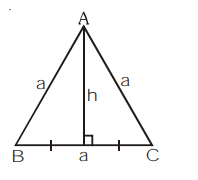# In an equailateral triangle,`
Question.

In an equailateral triangle, prove that three times the square of one side is equal to four times the square of one of its altitudes.

Solution:Altitude of equilateral $\Delta=\frac{\sqrt{3}}{2}$ side

$h=\frac{\sqrt{3}}{2} a$

$h^{2}=\frac{3}{4} a^{2}$

$4 h^{2}=3 a^{2}$